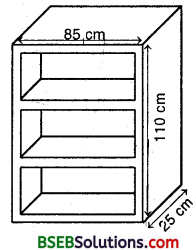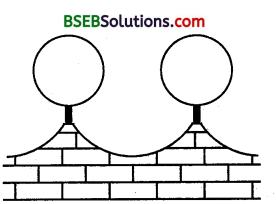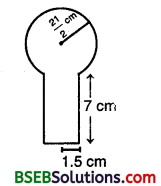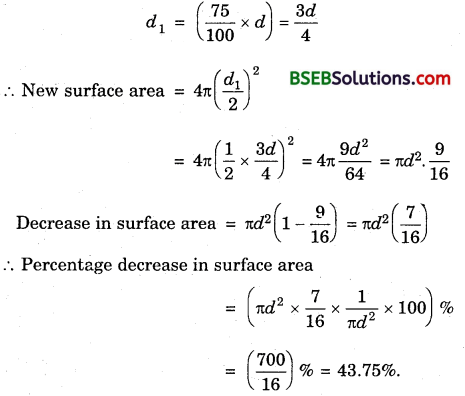# HSSlive: Plus One & Plus Two Notes & Solutions for Kerala State Board

## BSEB Class 9 Maths Chapter 13 Surface Areas and Volumes Ex 13.9 Textbook Solutions PDF: Download Bihar Board STD 9th Maths Chapter 13 Surface Areas and Volumes Ex 13.9 Book AnswersBSEB Class 9 Maths Chapter 13 Surface Areas and Volumes Ex 13.9 Textbook Solutions PDF: Download Bihar Board STD 9th Maths Chapter 13 Surface Areas and Volumes Ex 13.9 Book Answers

BSEB Class 9th Maths Chapter 13 Surface Areas and Volumes Ex 13.9 Textbooks Solutions and answers for students are now available in pdf format. Bihar Board Class 9th Maths Chapter 13 Surface Areas and Volumes Ex 13.9 Book answers and solutions are one of the most important study materials for any student. The Bihar Board Class 9th Maths Chapter 13 Surface Areas and Volumes Ex 13.9 books are published by the Bihar Board Publishers. These Bihar Board Class 9th Maths Chapter 13 Surface Areas and Volumes Ex 13.9 textbooks are prepared by a group of expert faculty members. Students can download these BSEB STD 9th Maths Chapter 13 Surface Areas and Volumes Ex 13.9 book solutions pdf online from this page.

## Bihar Board Class 9th Maths Chapter 13 Surface Areas and Volumes Ex 13.9 Books Solutions

 Board BSEB Materials Textbook Solutions/Guide Format DOC/PDF Class 9th Subject Maths Chapter 13 Surface Areas and Volumes Ex 13.9 Chapters All Provider Hsslive

## How to download Bihar Board Class 9th Maths Chapter 13 Surface Areas and Volumes Ex 13.9 Textbook Solutions Answers PDF Online?

2. Click on the Bihar Board Class 9th Maths Chapter 13 Surface Areas and Volumes Ex 13.9 Answers.
3. Look for your Bihar Board STD 9th Maths Chapter 13 Surface Areas and Volumes Ex 13.9 Textbooks PDF.
4. Now download or read the Bihar Board Class 9th Maths Chapter 13 Surface Areas and Volumes Ex 13.9 Textbook Solutions for PDF Free.

## BSEB Class 9th Maths Chapter 13 Surface Areas and Volumes Ex 13.9 Textbooks Solutions with Answer PDF Download

Find below the list of all BSEB Class 9th Maths Chapter 13 Surface Areas and Volumes Ex 13.9 Textbook Solutions for PDF’s for you to download and prepare for the upcoming exams:

## BSEB Bihar Board Class 9th Maths Solutions Chapter 13 Surface Areas and Volumes Ex 13.9

Question 1.
A wooden bookshelf has external dimensions as follows : Height = 110 cm, Depth = 25 cm, Breadth 85 cm (see figure). The thickness of the plank is 5 cm everywhere. The external faces are to be polished and the inner faces are to be painted. If the rate of polishing is 20 paise per cm² and the rate of painting is 10 paise per cm², find the total expenses required for polishing and painting the surface of the bookshelf.Solution:
Area to be polished
= (110 x 85 + 2 x 85 x 25 + 2 x 25 x 110 + 4 x 75 x 5 + 2 x 110 x 5) cm²
= (9350 + 4250 + 5500 + 1500 + 1100) cm²
= 21700 cm²
Cost of polishing @ 20 paise per cm²
= (21700 x 20100)
= Rs 4340
Area to be painted
= (6 x 75 x 20 + 2 x 90 x 20 + 75 x 90) cm²
= (9000 + 3600 + 6750) cm² = 19350 cm²
Cost of painting @ 10 paise per cm²
= Rs [ 19350 x 10100] = Rs 1935
∴ Total expenses = Rs (4340 + 1935) = Rs 6275

Question 2.
The front compound wall of a house is decorated by wooden spheres of diameter 21 cm, placed on small supports as shown in figure. Eight such spheres are used for this purpose, and are to be painted silver. Each support is a cylinder of radius 1.5 cm and . height 7 cm and is to be painted black. Find the cost of paint required if silver paint costs 25 paise per cm² and black paint costs 5 paise per cm².Solution:
Clearly, we have to subtract the part of the sphere that is resting on the sphere while calculating the cost of silver paint.
Surface area to be silver paint
= 8 (Curved surface area of the sphere – area of circle on which sphere is resting)
= 8(4πR² – πr²) cm², where R = 212 cm, r = 1.5 cm
= 8π(4 x 4414 – 2.25) cm²
= 8π(441 – 2.25) cm²
= 8π(438.75) cm²
∴ Cost of silver paint @ 25 paise per cm²= Rs (8 x 227 x 438.75 x 25100]
= Rs(193057) = Rs 2757.86 (approx.)
Surface area to be black painted
= 8 x curved surface area of cylinder
= 8 x 2πrh = 8 x 2 x 227 x 1.5 x 7 cm²
= 528 cm²
Cost of black paint @ 5 paise per cm² = Rs (528 x 5100) = RS 26.40
Total cost of painting = Rs (2757.86 + 26.40) = Rs 2784.26 (appiox)

Question 3.
The diameter of a sphere is decreased by 25%. By what percent does its curved surface area decrease?
Solution:
Let d be the diameter of the sphere.
Then, its surface area = 4π(𝑑2)² = πd²
On decreasing its diameter by 25%, the new diameter,## Bihar Board Class 9th Maths Chapter 13 Surface Areas and Volumes Ex 13.9 Textbooks for Exam Preparations

Bihar Board Class 9th Maths Chapter 13 Surface Areas and Volumes Ex 13.9 Textbook Solutions can be of great help in your Bihar Board Class 9th Maths Chapter 13 Surface Areas and Volumes Ex 13.9 exam preparation. The BSEB STD 9th Maths Chapter 13 Surface Areas and Volumes Ex 13.9 Textbooks study material, used with the English medium textbooks, can help you complete the entire Class 9th Maths Chapter 13 Surface Areas and Volumes Ex 13.9 Books State Board syllabus with maximum efficiency.

## FAQs Regarding Bihar Board Class 9th Maths Chapter 13 Surface Areas and Volumes Ex 13.9 Textbook Solutions

#### How to get BSEB Class 9th Maths Chapter 13 Surface Areas and Volumes Ex 13.9 Textbook Answers??

Students can download the Bihar Board Class 9 Maths Chapter 13 Surface Areas and Volumes Ex 13.9 Answers PDF from the links provided above.

#### Can we get a Bihar Board Book PDF for all Classes?

Yes you can get Bihar Board Text Book PDF for all classes using the links provided in the above article.

## Important Terms

Bihar Board Class 9th Maths Chapter 13 Surface Areas and Volumes Ex 13.9, BSEB Class 9th Maths Chapter 13 Surface Areas and Volumes Ex 13.9 Textbooks, Bihar Board Class 9th Maths Chapter 13 Surface Areas and Volumes Ex 13.9, Bihar Board Class 9th Maths Chapter 13 Surface Areas and Volumes Ex 13.9 Textbook solutions, BSEB Class 9th Maths Chapter 13 Surface Areas and Volumes Ex 13.9 Textbooks Solutions, Bihar Board STD 9th Maths Chapter 13 Surface Areas and Volumes Ex 13.9, BSEB STD 9th Maths Chapter 13 Surface Areas and Volumes Ex 13.9 Textbooks, Bihar Board STD 9th Maths Chapter 13 Surface Areas and Volumes Ex 13.9, Bihar Board STD 9th Maths Chapter 13 Surface Areas and Volumes Ex 13.9 Textbook solutions, BSEB STD 9th Maths Chapter 13 Surface Areas and Volumes Ex 13.9 Textbooks Solutions,
Share: